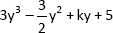# Class 9 Polynomials Important Questions

Class 9 Polynomials Important Questions

1. Using suitable identity determine the value of :-
1. (17)3 + (-12)3 + (-5)3
2. 64m3 – 343n3
2. Factorise :-
1. 2y3 – 4y2 – 2y + 4
2. 2x2 + 7x + 3
3. Factorise : (a-b)3 + (b-c)3 + (c-a)3
4. If (a + b + c) = 15 and a2 + b2 + c2 = 83, find the value of a3 + b3 + c3 – 3abc.
5. If x3 + ax2 – bx + 10 is divisible by x2 – 3x + 2, find the values of a and b.
6. Verify that x3 + y3 + z3 – 3xyz = ½ (x + y + z)[(x – y)2 + (y – z)2 + (z – x)2]
7. Find the value of k for which the cubic polynomialis exactly divisible by y – ½.
8. Prove that (a + b + c)3a3b3 c3 = 3(a + b)(b + c)(c + a)
9. If x – 3 and x – 1/3 are both factors of px2 + 5x + r, then show that p = r.
10. If x2 – 1 is a factor of ax4 + bx3 + cx2 + dx + e, show that a + c + e = b + d = 0.
11. If a + b + c = 0, find (a2/bc + b2/ca + c2/ab) .